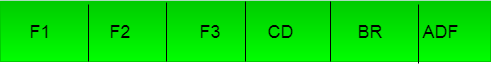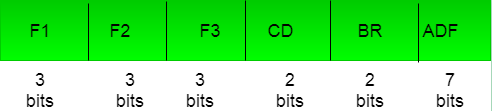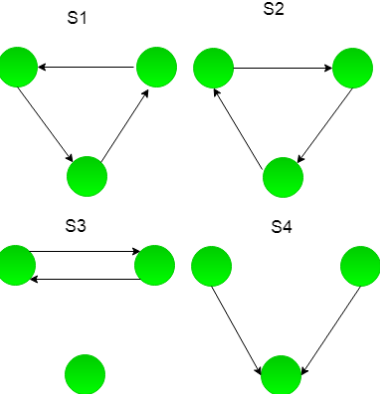# UGC NET COMPUTER SCIENCE SOLVED PAPERS 2017-19 - UGC NET Computer Science November 2017 Paper 3

>>>>>>>>UGC NET Computer Science November 2017 Paper 3

• A

17• B

20• C

24• D

32• Option : B
• Explanation :
Microprocessor instruction format, which is divided into three subfields F1, F2, F3 each having seven distinct micro-operations, condition field CD for four status bits, branch field BR having four options used in conjunction with address field ADF. The address space is of 128 memory locations.ie:F1,F2,F3 each having seven distinct micro-operation. So, 3 bits are required for each. Condition field have four status, it needs 2 bits for four different condition. Branch field have four option so,it needs 2 bits for four option. Now there are 128 different memory location, So, there 7 bits atre required for 128 diffeent location. Instruction Field:Total bits are 20. So, option (B) is correct.

• A

S1• B

S2• C

S3• D

S4• Option : D
• Explanation :
We can draw precedence graph for each schedule and for conflict serializability graph must not contain cycle.So, option (D) is correct.

• A

Topological order• B

Depth - first order• C• D

Ascending order of transaction indices• Option : A
• Explanation :
For a schedule, we can check its serializability by drawing a precedence graph and find its topological order, precedence graph of schedule must not contain any cycle to be conflict free. Refer:GATE-CS-2016 So, option (A) is correct.

• A

First normal form• B

Second normal form• C

Third normal form• D

Fourth normal form• Option : B
• Explanation :
Conditions for various normal forms:
1. 1 NF - A relation R is in first normal form (1NF) if and only if all underlying domains contain atomic values only.
2. 2 NF - A relation R is in second normal form (2NF) if and only if it is in 1NF and every non-key attribute is fully dependent on the primary key.
3. 3 NF - A relation R is in third normal form (3NF) if and only if it is in 2NF and every non-key attribute is non-transitively dependent on the primary key.
4. BCNF - A relation R is in Boyce-Codd normal form (BCNF) if and only if every determinant is a candidate key.

Example: Relation R(XYZ) with functional dependencies {X -> Y, Y -> Z, X -> Z}. Notice here Y -> Z, in question it is not mention that non prime attribute is only dependent on primary key so this FD is perfectly valid. This relation is in 2NF but not in 3NF because of every non-key attribute is transitively dependent on the primary key. Here {X} will be candidate key. So, option (B) is correct.

• A

in 1NF but not in 2NF• B

in 2NF but not in 3NF• C

in 3NF but not in BCNF• D

in BCNF• Option : A
• Explanation :
If we find closure of A: A+ → All atribute except D. Similarly for other keys we can find closure, but D can't be derived from any key and it must be added to all keys to be derived from. That's why this relation is in 1NF, since there is partial dependency so, this relation is not in 2NF. So, option (A) is correct